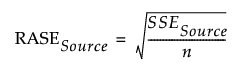Predictive and Specialized Modeling > Model Screening > The Model Screening Report > Training, Validation, and Test Measures of Fit
Publication date: 05/24/2021

## Training, Validation, and Test Measures of Fit

There is a measures of fit report for each model data set that is specified. These could be Training, Training and Validation, or Training, Validation, and Test sets. Each report contains a table with the following columns:

Method

The name of the method used to fit the model.

N

The number of observations in the set.

Sum Wgt

The sum of the weights.

RSquare

(Available only for continuous responses.) The RSquare value of the fitted model.

Entropy RSquare

(Available only for categorical responses.) A measure of fit that compares the log-likelihoods from the fitted model and the constant probability model. Entropy RSquare ranges from 0 to 1, where values closer to 1 indicate a better fit. See Entropy RSquare.

Misclassification Rate

(Available only for categorical responses.) The proportion of observations misclassified by the model. Smaller values indicate a better fit.

Note: In these tables, the misclassification rate is always calculated using a probability threshold of 0.5.

AUC

(Available only for categorical responses.) The area under the ROC curve. Values closer to 1 indicate a better fit.

RASE

The square root of the mean squared prediction error (Root Average Square Error). RASE is computed as follows, where Source indicates the Training, Validation, or Test set.Generalized RSquare

(Available only for categorical responses.) A measure that can be applied to general regression models. It is based on the likelihood function L and is scaled to have a maximum value of 1. The value is 1 for a perfect model, and 0 for a model no better than a constant model. The Generalized RSquare measure simplifies to the traditional RSquare for continuous normal responses in the standard least squares setting. Generalized RSquare is also known as the Nagelkerke or Craig and Uhler R2, which is a normalized version of Cox and Snell’s pseudo R2. See Nagelkerke (1991).

Fold

(Available only if the K Fold Crossvalidation option or the Nested Crossvalidation option is specified in the launch window.) Identifies the fold that is held out for the model fit in that row.

Inner Fold

(Available only if the Nested Crossvalidation option is specified in the launch window.) Identifies the inner fold that is held out for the model fit in that row.

Trial

(Available only if the Repeated K Fold option is specified in the launch window.) Identifies the trial number for the model fit in that row.

The following options are available below each table:

Select Dominant

Selects each model that is better than or equal to all of the other models in terms of a combination of model fitting criteria. For continuous responses, RSquare and Sum Freq are considered when determining the dominant model. For categorical responses, Entropy RSquare, Misclassification Rate, AUC, and Sum Freq are considered when determining the dominant model.

Run Selected

Runs the individual models specified in each selected row.

Save Script Selected

Saves a model script to the script window for each selected row.